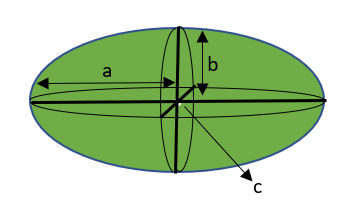# How to calculate the Volume of an Ellipsoid?

• Last Updated : 10 Feb, 2022

Mensuration is a sub-branch of mathematics that is used to discuss different types of geometrical shapes like cube, cylinder, ellipsoid, their areas, and volume. It is used to find the geometric and algebraic equations that are used to measure various aspects of objects or shapes like Surface Area, Volume, Curve Surface area, etc. In mensuration, volume is used to calculate the amount of space inside a 3D object that can be filled. We can find the volume of any solid object like square, cylinder, ellipsoid, etc. In this article, we will learn how to calculate the volume of the Ellipsoid.

Ellipsoid

Ellipsoid is a three-dimensional geometric figure. It is a closed surface in an elliptical shape and is seen as a structured ellipse. It gets its name from the ellipse because any plane that cuts through the ellipsoid will form an ellipse. It has three axes of rotational symmetry and these three axes are perpendicular to each other and meet at one point which is known as the center of the ellipsoid.  Ellipsoid are of two types:

• Oblate Ellipsoid: If a = b and a > c, then such type of ellipsoid is known as Oblate ellipsoid.
• Prolate Ellipsoid: If a = b and c > a, then such type of ellipsoid is known as a prolate ellipsoid.

The standard equation of ellipsoid is

x2/a2 + y2/b2 + z2/c2 = 1

Here a ≠ b ≠ c. If a = b = c then that ellipsoid is known as a sphere.

### Volume of the Ellipsoid

The volume of the ellipsoid is the measurement of the ellipsoid that expresses the amount of three-dimensional space enclosed by a closed surface.As we know that the equation of ellipsoid be (x2/a2) + (y2/b2) + (z2/c2) = 1 where a, b, c are the lengths of semi-axes of ellipsoid then volume can be calculated by the below formula-

Volume of Ellipsoid = (4/3) × π × a × b × c

The volume of Oblate Ellipsoid is

Volume of Oblate Ellipsoid = (4/3) × π × a × a × b

The volume of Prolate Ellipsoid is

Volume of Prolate Ellipsoid = (4/3) × π × a × b × b

Example:

Given the length of semi-axes are 5cm, 6cm, 4cm

So the volume of the ellipsoid is

V = (4/3) × π × a × b × c

= (4/3) × π × 5 × 6 × 4

= 430/3

= 160

Hence the volume of the ellipsoid is 160

Determining the volume of the ellipsoid

As we know that the equation of ellipsoid is

(x2/a2) + (y2/b2) + (z2/c2) = 1

Let us assume that -a ≤ x ≤ a

Now, we cut the ellipsoid with a plane parallel to the yz-plane

So, we get an ellipse

(y2/b2) + (z2/c2) = 1 – (x2/a2

(y2/b2(1 – (x2/a2))) + (z2/c2(1 – (x2/a2) )) = 1

So the semiaxes are

p = b√(1 – (x2/a2)) and q = c√(1 – (x2/a2))

As we know that the area of ellipse is

A(x) =  πbc(1 – (x2/a2)) …..(1)

Now by using the formula of parent entry we calculate the volume of the ellipsoid

V = \limiNow put the value of A(x) fro equation (1), we get

V = πbc \limiV = 4/3πbc

### Sample Questions

Question 1: Find the volume of the ellipsoid if the lengths of semi-axes are 3cm, 4cm, 2cm.

Solution:

Given,

Lengths of semi axes of an ellipsoid a=3cm, b=4cm, c=2cm

Volume = (4/3) × π × a × b × c

= (4/3) × π × 3 × 4 × 2

= 32 × π

= 100.53 cm3

So, volume of ellipsoid with given measurements is 100.53cm3.

Question 2: Find the volume of the ellipsoid if the lengths of semi-axes are 5cm, 3cm, 2cm.

Solution:

Given,

Lengths of semi axes of an ellipsoid a = 5cm, b = 3cm, c = 2cm

Volume = (4/3) × π × a × b × c

= (4/3) × π × 5 × 3 × 2

= 40 × π

= 125.66 cm3

So, volume of ellipsoid with given measurements is 125.66cm3.

Question 3: Find the volume of the ellipsoid if the lengths of axes are 6cm, 4cm, 2cm.

Solution:

Given,

Lengths of axes of an ellipsoid are 6cm, 4cm and 2cm.

Length of semi axes = Length of axes/2

a = (6/2) = 3cm

b = (4/2) = 2cm

c = (2/2) = 1cm

Volume = (4/3) × π × a × b × c

= (4/3) × π × 3 × 2 × 1

= 8× π

= 25.13 cm3

So, volume of ellipsoid with given measurements is 25.13cm3.

Question 4: Find the volume of the ellipsoid if the lengths of axes are 12cm, 6cm, and 2cm.

Solution:

Given,

Lengths of axes of an ellipsoid are 12cm, 6cm and 2cm.

Length of semi axes = Length of axes/2

a = (12/2) = 6cm

b = (6/2) = 3cm

c = (2/2) = 1cm

Volume = (4/3) × π × a × b × c

= (4/3) × π × 6 × 3 × 1

= 24× π

= 75.4 cm3

So, the volume of ellipsoid with given measurements is 75.4cm3.

Question 5: Find the volume of ellipsoid if the equation is given as (x2/72) + (y2/42) + (z2/22) = 1

Solution:

Given,

Equation of ellipsoid, (x2/72) + (y2/42) + (z2/22) = 1

It is of form (x2/a2) + (y2/b2) + (z2/c2) = 1

From this we can derive lengths of semi axes.

a = 7

b = 4

c = 2

Volume = (4/3) × π × a × b × c

= (4/3) × π × 7 × 4 × 2

= (224/3) × π

= 234.57 cm3

So, the volume of ellipsoid with given measurements is 234.57cm3.

My Personal Notes arrow_drop_up